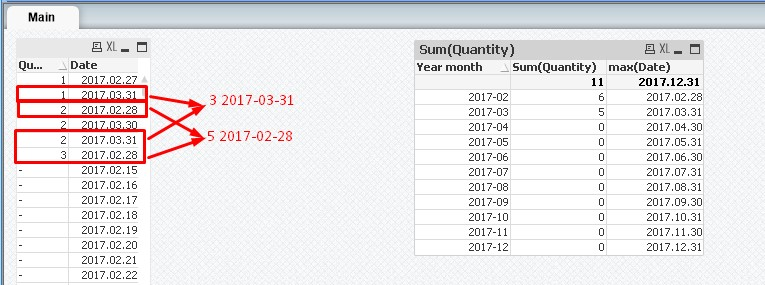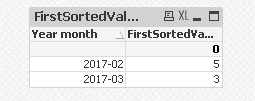# QlikView Scripting

Discussion Board for collaboration on QlikView Scripting.

Announcements
Coming your way, the Qlik Data Revolution Virtual Summit. October 27-29. REGISTER
cancel
Showing results for
Did you mean:
HighlightedSpecialist III

## Aggr or FirstSortedValue

Hello

I need some help.

I need to sum quantity according to the max date in the month:The result:

Year month, Sum

2017-02, 3

2017-03, 5

1 Solution

Accepted Solutions
HighlightedMVP

Try this:

FirstSortedValue(Aggr(Sum(Quantity), Date), -Date)9 Replies
HighlightedMVP

Try this:

FirstSortedValue(Aggr(Sum(Quantity), Date), -Date)HighlightedSpecialist

Try this,

Sum (Aggr (Firstsortedvalue (quantity,-Date),Year Month))

HighlightedSpecialist III

And how that would work in case we have two dimensions Year month, Product?

HighlightedMVP

Difficult to say without looking, but may be this:

FirstSortedValue(Aggr(Sum(Quantity), Product, Date), -Aggr(Date, Product, Date))

HighlightedSpecialist III

Thanks once again!

HighlightedNot applicable

Hi Sunny,

many thanks for your suggestion: it works very well! Instead, I have a problem when I try to apply the expression to calculate the previous year value.

In fact, it seems that the function FirstSortedValue is not able to consider a set analysis expression:

=sum(aggr(FirstSortedValue({<Date={'<=\$(=max(Date)-100)>=\$(=min(Date)-100)'}>}aggr(Costs,Project,Date),-aggr(Date,Project,Date)),Project))

The date format is YYYYMM (i.e. 201701)

Am I wrong? How can I manage this issue?

Best,

Matteo

HighlightedMVP

Not entirely sure, but try this

=Sum({<Date = {"\$(='<=' & Date(Max(Date)-100, 'YYYYMM') & '>=' & Date(Min(Date)-100, 'YYYYMM'))"}>} Aggr(FirstSortedValue({<Date = {"\$(='<=' & Date(Max(Date)-100, 'YYYYMM') & '>=' & Date(Min(Date)-100, 'YYYYMM'))"}>} Aggr(Only({<Date = {"\$(='<=' & Date(Max(Date)-100, 'YYYYMM') & '>=' & Date(Min(Date)-100, 'YYYYMM'))"}>} Costs), Project, Date), -Aggr(Only({<Date = {"\$(='<=' & Date(Max(Date)-100, 'YYYYMM') & '>=' & Date(Min(Date)-100, 'YYYYMM'))"}>} Date), Project, Date)), Project))

HighlightedNot applicable

Hi Sunny,

I implemented this formula because yours seems not to work in my dashboard:

=sum(aggr(FirstSortedValue({<Date={'<=\$(=max(Date)-100)>=\$(=min(Date)-100)'}>}aggr(only({<Date={'<=\$(=max(Date)-100)>=\$(=min(Date)-100)'}>} Costs),Project,Date),-aggr(only({<Date={'<=\$(=max(Date)-100)>=\$(=min(Date)-100)'}>}Date),Project,Date)),Project))

This one is fine on a local test qvw with a limited amount of data.

My current problem is to insert into this expression the sum function regarding Costs while keeping the set analysis for the previous year.

Do you have any idea on that?

Many thanks

HighlightedContributor II

Hi Sunny,

I need to calculate as of date figure for respective years and for previous years. For current years, the formula below is working:

FirstSortedValue(Aggr(Sum([Amount]), [Period]), -Aggr([Period], [Period]))

But I am struggling to calculate prior year figures using same. I have already tried for a week now but with no success. Can you help?